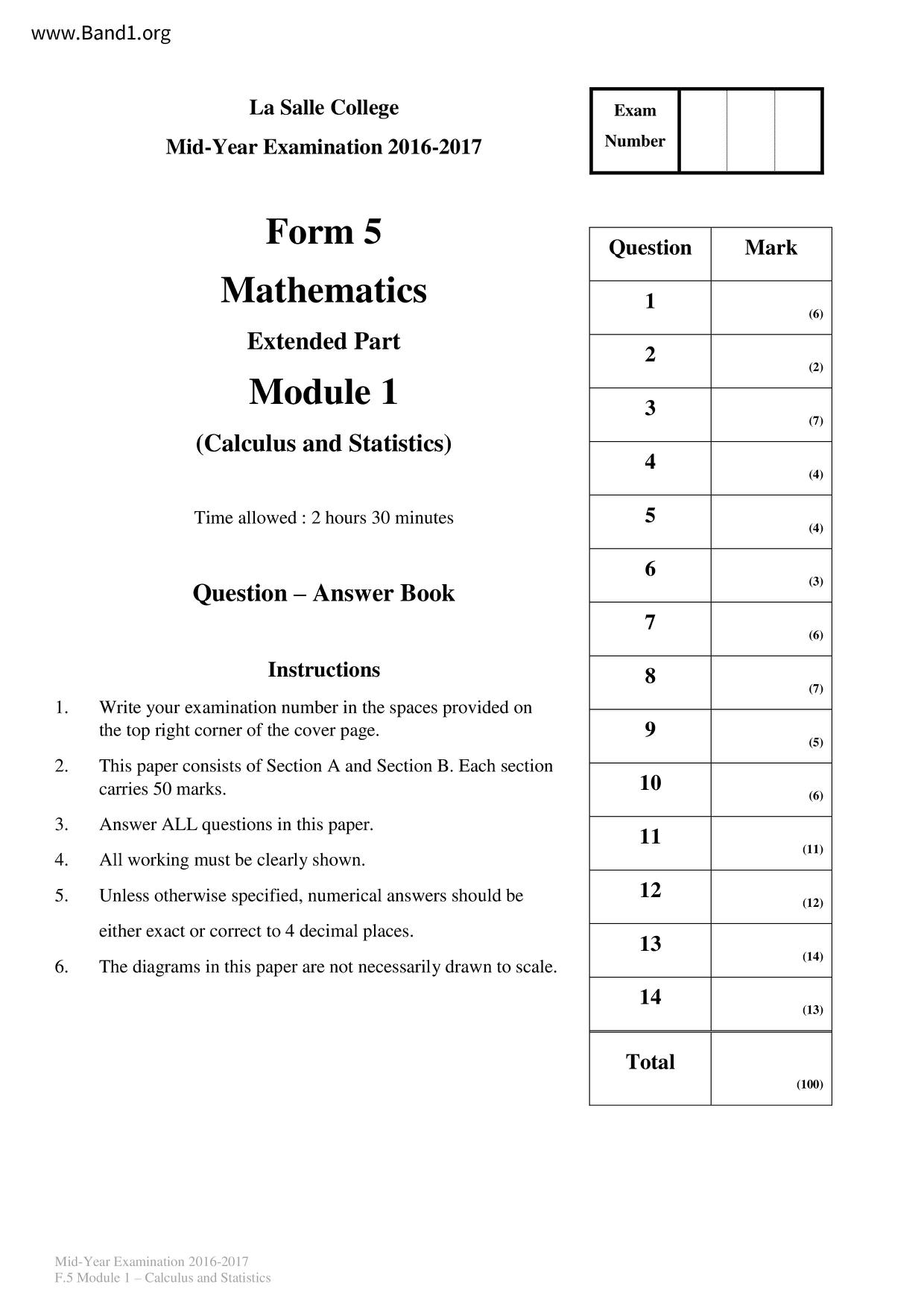# 中五 數學試卷 (F5 Maths Past Paper)

6632

pdf

20

F5 maths _MY_M1_16_17

▼ 圖片只作預覽, 如欲下載整份卷, 請按「免費成為會員」 ▼▲ 圖片只作預覽, 如欲下載整份卷, 請按「免費成為會員」 ▲

## 中五數學試卷 PDF 下載

La Salle College
Mid-Year Examination 2016-2017
Mathematics
Extended Part
(Calculus and Statistics)
Time allowed: 2 hours 30 minutes
Instructions
Write your examination number in the spaces provided on
the top right corner of the cover page.
This paper consists of Section A and Section B. Each section
carries 50 marks.
Answer ALL questions in this paper.
All working must be clearly shown.
Unless otherwise specified, numerical answers should be
either exact or correct to 4 decimal places.
The diagrams in this paper are not necessarily drawn to scale.
Mid-Year Examination 2016-2017
F.5 Module 1 - Calculus and Statistics
The curve y = x³ - 3x² - 10x+24 intersects the x-axis at points A, B and C. Find the shaded area
enclosed by the curve and the x-axis as shown in the figure.
Mid-Year Examination 2016-2017
and Statistics
y=x² − 3x² −10x + 24
(a) Show that [(x+1)+¹(x−1)″-¹] = 2(nx − 1)(x+1)″ (x−1)″-², where n is a constant.
Given that the slope at any point (x, y) of a curve C is given by
2(2017x-1)(x+1) 2017(x-1) 20¹5. If the y-intercept of C is 2, find the equation of C.
Mid-Year Examination 2016-2017
and Statistics
Section B [50 marks]
A researcher models the rate of change of antibodies in the bloodstream of a patient t hours after
injection of a vaccine by the following formula:
(a) Prove that A = 40ln
where A units is the amount of antibodies in the bloodstream. When t = 0, A = 300.
dt t² - 6t+10
Mid-Year Examination 2016-2017
and Statistics
t² - 6t+10
0≤t≤300,
Prove that t²-6t+10>0 for all real values of t.
When will the amount of antibodies in the bloodstream reach the minimum?
What is the minimum amount of antibodies in the bloodstream?
The patient will be protected when the amount of antibodies is not less than 500 units. The
researcher claims that the patient can be protected within 40 hours after the injection of the
Mid-Year Examination 2016-2017
and Statistics
The market research department of a smartphone manufacturer estimates the total sales (in
after t months of its launch,
thousands) of a new phone model will increase at a rate S'(t)=
where 0(a) The researcher uses the trapezoidal rule with 5 sub-intervals to estimate the total sales for the
first 5 months after its launch.
(i) Find the estimate.
The researcher re-estimates the total sales by expanding
powers of t.
Mid-Year Examination 2016-2017
and Statistics
in ascending powers of t as far as the term in 1³.
(ii) Using the result of (b)(i), re-estimate the total sales for the first 5 months after its
as a series in ascending
Mid-Year Examination 2016-2017
and Statistics
Let f(x)= x² Inx.
Find the range of value(s) of x such that f(x)≥0.
Using the result of (b)(i), or otherwise, show that
x + C, where C is a constant.
(x³ In x).
√ x² In x dx
(iii) Hence, show that the area bounded by the x-axis, the lines x = 1 and x = 2 as well as the
curve y = x ln x is
Mid-Year Examination 2016-2017
and Statistics
Using the results of (b)(iii) and (c)(i), show that
1.014 In 1.01 +1.024 In 1.02+1.034 In 1.03+...+In 1.994 In 1.99 > 632 ln 2-124.
Mid-Year Examination 2016-2017
and Statistics
A researcher models the number of people infected by bird flu x days after the outbreak of the
disease in a region with the following formula:
1+ a[e¹(x-1)]
where a and b are constants.
Mid-Year Examination 2016-2017
and Statistics
as a linear function of x.
It is found that the slope and the x-intercept are −3 and
Find a and b.
Show that y = P(x) is an increasing function.
(iii) Will the number of infected people exceed 500? Explain your answer.
+1 respectively.
Let a be the root of P"(x)=0. Find a.
Briefly describe the behaviour of P'(x) before and after x = a.
Mid-Year Examination 2016-2017
and Statistics
Section A [50 marks]
Solve the following equations.
(a) ex (e*+2)=8
(b) In(x+2)+In(x-1)=0
Mid-Year Examination 2016-2017
and Statistics
Mid-Year Examination 2016-2017
and Statistics
- End of Paper
2. Evaluate lim
Mid-Year Examination 2016-2017
and Statistics
In the expansion of
(a) Find the value of n.
Mid-Year Examination 2016-2017
and Statistics
Hence, find the constant term.
in ascending powers of x, the coefficient of the 3rd term is 420.
4. Find the equation of the tangent to the curve y = (x
y=(x² − 3)e¹-x at x=2.
Mid-Year Examination 2016-2017
and Statistics
It is given that f(x)= x³ − 2x² − 4x+5. Find the range of values of x such that the curve
y = f(x) is
(a) decreasing;
(b) concave upwards.
Mid-Year Examination 2016-2017
and Statistics
Anson deposited a certain amount of money in a bank at the beginning of 2016. The manager
claimed that Anson's profit, P(t) (in thousand dollars) after t months, followed the equation
= 3e 100. Find the profit obtained from March 2016 to August 2016, correct to the nearest
thousand dollars.
Mid-Year Examination 2016-2017
and Statistics
Adrian goes from Town A to Town B. He first rides on boat with a speed of 10 km/h to cross the
river to reach C and then rides on bicycle with a speed of 20 km/h to Town B as shown in the
figure. It is given that AD L BD with AD = 5 km and BD = 30 km. Let x km be the length of CD
and t hours be the time taken for Adrian to travel from Town A to Town B.
Prove that t=
Mid-Year Examination 2016-2017
and Statistics
2√x² +25-x+30
Adrian claims that he can travel from Town A to Town B within 2 hours. Is the claim correct?